Related Articles
Python | Pandas MultiIndex.from_product()
• Last Updated : 24 Dec, 2018

Python is a great language for doing data analysis, primarily because of the fantastic ecosystem of data-centric python packages. Pandas is one of those packages and makes importing and analyzing data much easier.

Pandas` MultiIndex.from_product()` function make a MultiIndex from the cartesian product of multiple iterables.

Syntax: MultiIndex.from_product(iterables, sortorder=None, names=None)

Parameters :
iterables : Each iterable has unique labels for each level of the index.
sortorder : Level of sortedness (must be lexicographically sorted by that level).
names : Names for the levels in the index.

Returns: index : MultiIndex

Example #1: Use `MultiIndex.from_product()` function to construct a MultiIndex from the cartesian product of multiple iterables.

 `# importing pandas as pd ` `import` `pandas as pd ` ` `  `# Create the first iterable ` `Price ``=``[``20``, ``35``, ``60``, ``85``] ` ` `  `# Create the second iterable ` `Name ``=``[``'Vanilla'``, ``'Strawberry'``] ` ` `  `# Print the first iterable ` `print``(Price) ` ` `  `# Print the second iterable ` `print``(``"\n"``, Name) `

Output :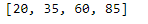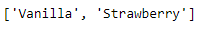Now let’s create the MultiIndex using the above two iterables.

 `# Creating the MultiIndex ` `midx ``=` `pd.MultiIndex.from_product([Name, Price], ` `                       ``names ``=``[``'Name'``, ``'Price'``]) ` ` `  `# Print the MultiIndex ` `print``(midx) `

Output :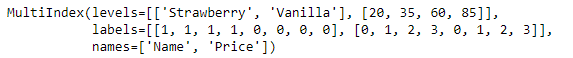As we can see in the output, the function has created a MultiIndex object using the cartesian product of these two iterables.

Example #2: Use `MultiIndex.from_product()` function to construct a MultiIndex from the cartesian product of multiple iterables.

 `# importing pandas as pd ` `import` `pandas as pd ` ` `  `# Create the first iterable ` `Snake ``=``[``'Viper'``, ``'Cobra'``] ` ` `  `# Create the second iterable ` `Variety ``=``[``'Brown'``, ``'Yellow'``, ``'Black'``] ` ` `  `# Print the first iterable ` `print``(Snake) ` ` `  `# Print the second iterable ` `print``(``"\n"``, Variety) `

Output :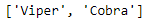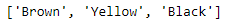Now let’s create the MultiIndex using the above two iterables.

 `# Creating the MultiIndex ` `midx ``=` `pd.MultiIndex.from_product([Snake, Variety],  ` `                       ``names ``=``[``'Snake'``, ``'Variety'``]) ` ` `  `# Print the MultiIndex ` `print``(midx) `

Output :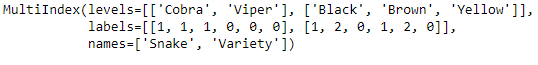The function has created a MultiIndex using the two iterables.

Attention geek! Strengthen your foundations with the Python Programming Foundation Course and learn the basics.

To begin with, your interview preparations Enhance your Data Structures concepts with the Python DS Course.

My Personal Notes arrow_drop_up
Recommended Articles
Page :